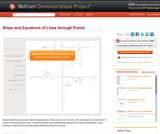# 1 Result

View
Selected filters:
• Tom FalconeConditions of Use:
يمكن إعادة ترتيب المحتوى ومشاركته
Rating

Algebra students need practice determining equations of lines given a pair of points, or the line parallel or perpendicular to a given line through a given point. This Demonstration, along with guiding worksheets or a teacher presentation, gives students a chance to see the relationships between these lines and points.

نوع المادة:
Activity/Lab
Diagram/Illustration
Homework/Assignment
تفاعلية
محاكاة
Teaching/Learning Strategy
Provider:
Wolfram Research
Provider Set:
Wolfram Demonstrations Project
المؤلف:
Tom Falcone